Home » A Practical Introduction to K-Nearest Neighbors Algorithm for Regression (with Python code)

# A Practical Introduction to K-Nearest Neighbors Algorithm for Regression (with Python code)

## Introduction

Out of all the machine learning algorithms I have come across, KNN algorithm has easily been the simplest to pick up. Despite its simplicity, it has proven to be incredibly effective at certain tasks (as you will see in this article).

And even better? It can be used for both classification and regression problems! KNN algorithm is by far more popularly used for classification problems, however. I have seldom seen KNN being implemented on any regression task. My aim here is to illustrate and emphasize how KNN can be equally effective when the target variable is continuous in nature.

If you want to understand KNN algorithm in a course format, here is the link to our free course- K-Nearest Neighbors (KNN) Algorithm in Python and R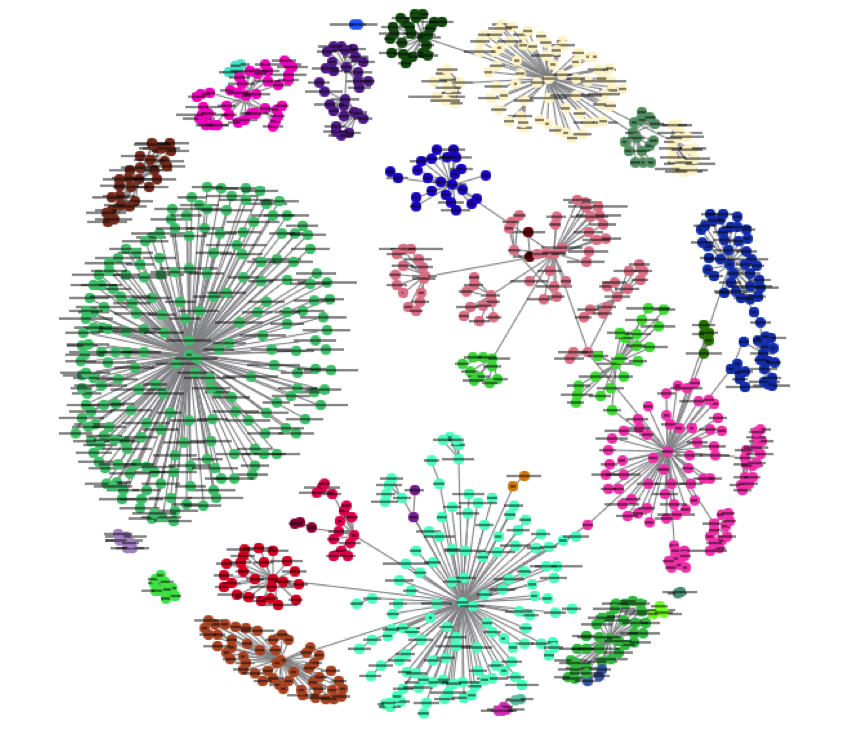In this article, we will first understand the intuition behind KNN algorithms, look at the different ways to calculate distances between points, and then finally implement the algorithm in Python on the Big Mart Sales dataset. Let’s go!

Note: Here is a link to understand KNN in a more structured format using our free course:

1. A simple example to understand the intuition behind KNN algorithm
2. How does the KNN algorithm work?
3. Methods of calculating the distance between points
4. How to choose the k factor?
5. Working on a dataset

## 1. A simple example to understand the intuition behind KNN

Let us start with a simple example. Consider the following table – it consists of the height, age and weight (target) value for 10 people. As you can see, the weight value of ID11 is missing. We need to predict the weight of this person based on their height and age.

Note: The data in this table does not represent actual values. It is merely used as an example to explain this concept.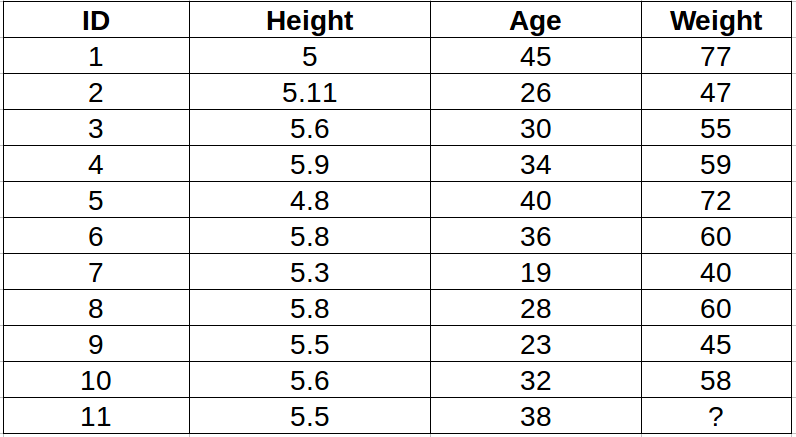For a clearer understanding of this, below is the plot of height versus age from the above table: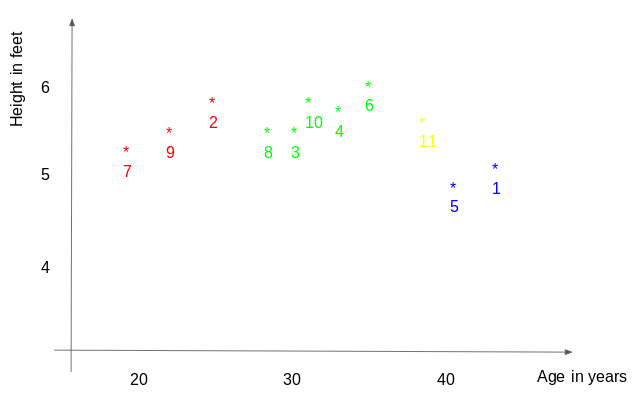In the above graph, the y-axis represents the height of a person (in feet) and the x-axis represents the age (in years). The points are numbered according to the ID values. The yellow point (ID 11) is our test point.

If I ask you to identify the weight of ID11 based on the plot, what would be your answer? You would likely say that since ID11 is closer to points 5 and 1, so it must have a weight similar to these IDs, probably between 72-77 kgs (weights of ID1 and ID5 from the table). That actually makes sense, but how do you think the algorithm predicts the values? We will find that out in this article.

Here is a free video-based course to help you understand KNN algorithm – K-Nearest Neighbors (KNN) Algorithm in Python and R

## 2. How does the KNN algorithm work?

As we saw above, KNN algorithm can be used for both classification and regression problems. The KNN algorithm uses ‘feature similarity’ to predict the values of any new data points. This means that the new point is assigned a value based on how closely it resembles the points in the training set. From our example, we know that ID11 has height and age similar to ID1 and ID5, so the weight would also approximately be the same.

Had it been a classification problem, we would have taken the mode as the final prediction. In this case, we have two values of weight – 72 and 77. Any guesses on how the final value will be calculated? The average of the values is taken to be the final prediction.

Below is a stepwise explanation of the algorithm:

1. First, the distance between the new point and each training point is calculated.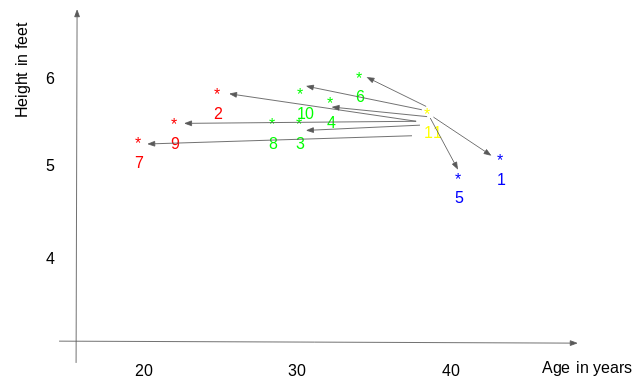2. The closest k data points are selected (based on the distance). In this example, points 1, 5, 6 will be selected if the value of k is 3. We will further explore the method to select the right value of k later in this article.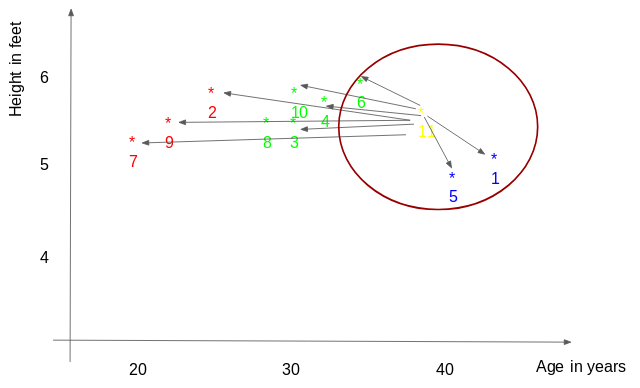3. The average of these data points is the final prediction for the new point. Here, we have weight of ID11 = (77+72+60)/3 = 69.66 kg.

In the next few sections, we will discuss each of these three steps in detail.

## 3. Methods of the calculating distance between points

The first step is to calculate the distance between the new point and each training point. There are various methods for calculating this distance, of which the most commonly known methods are – Euclidian, Manhattan (for continuous) and Hamming distance (for categorical).

1. Euclidean Distance: Euclidean distance is calculated as the square root of the sum of the squared differences between a new point (x) and an existing point (y).
2. Manhattan Distance: This is the distance between real vectors using the sum of their absolute difference.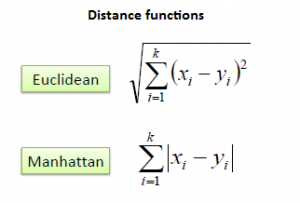3. Hamming Distance: It is used for categorical variables. If the value (x) and the value (y) are the same, the distance D will be equal to 0 . Otherwise D=1.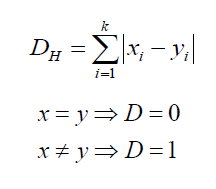Once the distance of a new observation from the points in our training set has been measured, the next step is to pick the closest points. The number of points to be considered is defined by the value of k.

## 4. How to choose the k factor?

The second step is to select the k value. This determines the number of neighbors we look at when we assign a value to any new observation.

In our example, for a value k = 3, the closest points are ID1, ID5 and ID6.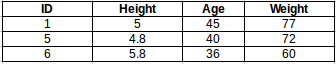The prediction of weight for ID11 will be:

```ID11 = (77+72+60)/3

ID11 = 69.66 kg```

For the value of k=5, the closest point will be ID1, ID4, ID5, ID6, ID10.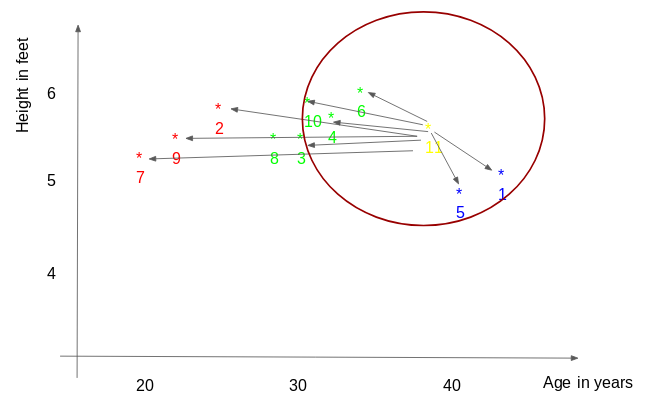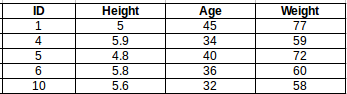The prediction for ID11 will be :

```ID 11 =  (77+59+72+60+58)/5

ID 11 = 65.2 kg```

We notice that based on the k value, the final result tends to change. Then how can we figure out the optimum value of k? Let us decide it based on the error calculation for our train and validation set (after all, minimizing the error is our final goal!).

Have a look at the below graphs for training error and validation error for different values of k.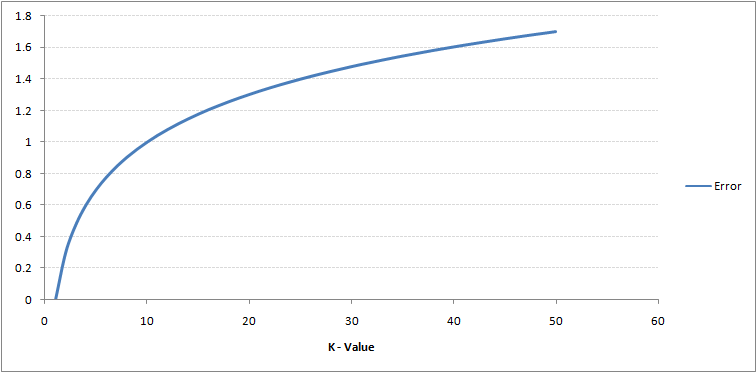For a very low value of k (suppose k=1), the model overfits on the training data, which leads to a high error rate on the validation set. On the other hand, for a high value of k, the model performs poorly on both train and validation set. If you observe closely, the validation error curve reaches a minima at a value of k = 9. This value of k is the optimum value of the model (it will vary for different datasets). This curve is known as an ‘elbow curve‘ (because it has a shape like an elbow) and is usually used to determine the k value.

You can also use the grid search technique to find the best k value. We will implement this in the next section.

## 5. Work on a dataset (Python codes)

By now you must have a clear understanding of the algorithm. If you have any questions regarding the same, please use the comments section below and I will be happy to answer them. We will now go ahead and implement the algorithm on a dataset. I have used the Big Mart sales dataset to show the implementation and you can download it from this link.

The full Python code is below but we have a really cool coding window here where you can code your own k-Nearest Neighbor model in Python:

```import pandas as pd

2. Impute missing values

```df.isnull().sum()
#missing values in Item_weight and Outlet_size needs to be imputed
mean = df['Item_Weight'].mean() #imputing item_weight with mean
df['Item_Weight'].fillna(mean, inplace =True)

mode = df['Outlet_Size'].mode() #imputing outlet size with mode
df['Outlet_Size'].fillna(mode, inplace =True)```

3. Deal with categorical variables and drop the id columns

```df.drop(['Item_Identifier', 'Outlet_Identifier'], axis=1, inplace=True)
df = pd.get_dummies(df)```

4. Create train and test set

```from sklearn.model_selection import train_test_split
train , test = train_test_split(df, test_size = 0.3)

x_train = train.drop('Item_Outlet_Sales', axis=1)
y_train = train['Item_Outlet_Sales']

x_test = test.drop('Item_Outlet_Sales', axis = 1)
y_test = test['Item_Outlet_Sales']```

5. Preprocessing – Scaling the features

```from sklearn.preprocessing import MinMaxScaler
scaler = MinMaxScaler(feature_range=(0, 1))

x_train_scaled = scaler.fit_transform(x_train)
x_train = pd.DataFrame(x_train_scaled)

x_test_scaled = scaler.fit_transform(x_test)
x_test = pd.DataFrame(x_test_scaled)```

6. Let us have a look at the error rate for different k values

```#import required packages
from sklearn import neighbors
from sklearn.metrics import mean_squared_error
from math import sqrt
import matplotlib.pyplot as plt
%matplotlib inline
```
```rmse_val = [] #to store rmse values for different k
for K in range(20):
K = K+1
model = neighbors.KNeighborsRegressor(n_neighbors = K)

model.fit(x_train, y_train)  #fit the model
pred=model.predict(x_test) #make prediction on test set
error = sqrt(mean_squared_error(y_test,pred)) #calculate rmse
rmse_val.append(error) #store rmse values
print('RMSE value for k= ' , K , 'is:', error)
```

Output :

```RMSE value for k = 1 is: 1579.8352322344945
RMSE value for k = 2 is: 1362.7748806138618
RMSE value for k = 3 is: 1278.868577489459
RMSE value for k = 4 is: 1249.338516122638
RMSE value for k = 5 is: 1235.4514224035129
RMSE value for k = 6 is: 1233.2711649472913
RMSE value for k = 7 is: 1219.0633086651026
RMSE value for k = 8 is: 1222.244674933665
RMSE value for k = 9 is: 1219.5895059285074
RMSE value for k = 10 is: 1225.106137547365
RMSE value for k = 11 is: 1229.540283771085
RMSE value for k = 12 is: 1239.1504407152086
RMSE value for k = 13 is: 1242.3726040709887
RMSE value for k = 14 is: 1251.505810196545
RMSE value for k = 15 is: 1253.190119191363
RMSE value for k = 16 is: 1258.802262564038
RMSE value for k = 17 is: 1260.884931441893
RMSE value for k = 18 is: 1265.5133661294733
RMSE value for k = 19 is: 1269.619416217394
RMSE value for k = 20 is: 1272.10881411344```

```#plotting the rmse values against k values
curve = pd.DataFrame(rmse_val) #elbow curve
curve.plot()```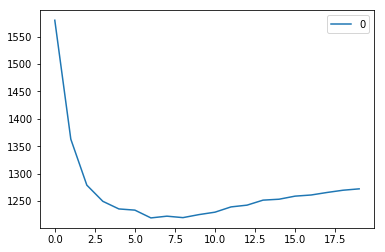As we discussed, when we take k=1, we get a very high RMSE value. The RMSE value decreases as we increase the k value. At k= 7, the RMSE is approximately 1219.06, and shoots up on further increasing the k value. We can safely say that k=7 will give us the best result in this case.

These are the predictions using our training dataset. Let us now predict the values for test dataset and make a submission.

7. Predictions on the test dataset

```#reading test and submission files
submission['Item_Identifier'] = test['Item_Identifier']
submission['Outlet_Identifier'] = test['Outlet_Identifier']

#preprocessing test dataset
test.drop(['Item_Identifier', 'Outlet_Identifier'], axis=1, inplace=True)
test['Item_Weight'].fillna(mean, inplace =True)
test = pd.get_dummies(test)
test_scaled = scaler.fit_transform(test)
test = pd.DataFrame(test_scaled)

#predicting on the test set and creating submission file
predict = model.predict(test)
submission['Item_Outlet_Sales'] = predict
submission.to_csv('submit_file.csv',index=False)

```

On submitting this file, I get an RMSE of 1279.5159651297.

8. Implementing GridsearchCV

For deciding the value of k, plotting the elbow curve every time is be a cumbersome and tedious process. You can simply use gridsearch to find the best value.

```from sklearn.model_selection import GridSearchCV
params = {'n_neighbors':[2,3,4,5,6,7,8,9]}

knn = neighbors.KNeighborsRegressor()

model = GridSearchCV(knn, params, cv=5)
model.fit(x_train,y_train)
model.best_params_

```

Output :

`{'n_neighbors': 7}`

## 6. End Notes and additional resources

In this article, we covered the workings of the KNN algorithm and its implementation in Python. It’s one of the most basic, yet effective machine learning techniques. For KNN implementation in R, you can go through this article : kNN Algorithm using R. You can also go fou our free course – K-Nearest Neighbors (KNN) Algorithm in Python and R to further your foundations of KNN.

In this article, we used the KNN model directly from the sklearn library. You can also implement KNN from scratch (I recommend this!), which is covered in the this article: KNN simplified.

If you think you know KNN well and have a solid grasp on the technique, test your skills in this MCQ quiz: 30 questions on kNN Algorithm. Good luck!

You can also read this article on our Mobile APP•Paren Kansara says:

Hi Aishwarya, your explanation on KNN is really helpful. I have a doubt though. KNN suffers from the dimensionality curse i.e. Euclidean distance is not helpful when subjected to high dimensions as it is equidistant for different vectors.
What was your viewpoint while using the KNN despite this fact ? Curious to know. Thank you.

•Aishwarya Singh says:

Hi,

KNN works well for dataset with less number of features and fails to perform well has the number of inputs increase. Certainly other algorithms would show a better performance in that case. With this article I have tried to introduce the algorithm and explain how it actually works (instead of simply using it as a black box).

•Osman says:

Hi. I have been following you now for a while. Love your post. I wish you cold provide a pdf format also, because it is hard to archive and read web posts when you are offline.

•Aishwarya Singh says:

Hi Osman,

Really glad you liked the post. Although certain articles and cheat sheets are converted and shared as pdf, but not all articles are available in the format. Will certainly look into it and see if we can have an alternate.

•Abin Singh Rajan says:

Hi thanks for the explanations.

Can you explain the intuition behind categorical variable calculations.

For example, assume data set has height,age,gender as independent variable and weight as dependent variable.
Now if an unseen data comes with male as gender, then how it works? how it predicts the weight?

•Aishwarya Singh says:

Hi,

Excellent question!

Suppose we have gender as a feature, we would use hamming distance to find the closest point (We need to find the distance with each training point as discussed in the article). Let us take the first training point, if it has the gender male and my test point also has the gender male, then the distance D will be 0. Now we take the second training point, it it has gender female and the test point has gender male, the value of D will be 1. Simply put, we will consider the test point closer to first training point and predict the target value accordingly.

•Steffen says:

cannot find the data on https://datahack.analyticsvidhya.com/contest/practice-problem-big-mart-sales-iii/

•Aishwarya Singh says:

Hi Steffen,

Please register in the competition. Once you to that, you will be able to download the train, test and submission file.

•Paren Kansara says:

Hi Aishwarya, your explanation for KNN is very thorough and clear. I have a doubt though. KNN suffers from the dimensionality curse i.e. the Euclidean distance is equidistant for high dimensions and thus it is irrelevant. I am curious to know why you chose to use KNN despite this fact? Thank you.

•Aishwarya Singh says:

Hi,

I believe I have answered your question as a reply to your previous comment. Anyway, knn might not give the best set of predictions for this dataset but the idea was to introduce the algorithm and use a simple dataset to practice.

•Mukul MIshra says:

please provide the code for R as well

•Aishwarya Singh says:

Hi Mukul,

•Akshay says:

First of all, thank you for such a nice explanation.

I have couple of questions about the methods for calculating the distance.

1) Are Manhattan distance and Hamming Distance are same? Because by looking at the formulas, it looks like the same.

2) For Hamming Distance the article says ‘If the predicted value (x) and the real value (y) are same, the distance D will be equal to 0 . Otherwise D=1.” But the the formula itself will be use in the process of calculation of predicted value so how can we use the predicted value in Hamming Distance formula, I hope you got my question.

Thank you.

•Aishwarya Singh says:

Hi Akshay,

Manhattan distance and Hamming distance are not the same. Manhattan distance is for continuous variables while Hamming is used for categorical. I agree that the formula looks the same but the inputs (x and y) are not the same. Regarding the second question, x will be the values in the test set (for which you need to make predictions) and y will be the train set. So x and y are the features (here – the feature is gender), which means that we are not using the predicted values, but the features. Thanks for pointing it out, to avoid the confusion, I have updates the same in the article.

•Rajiv S says:

Hi Aishwarya,
This is the first time I have read one of your blogs. You are doing a fantastic job of explaining concepts in a lucid yet simple language. Keep it up!!!
It will help if you in the End notes section you can include limitations of the model usage like the one explained in response to Abin’s question.
Further would it be possible for you to publish a series on other models too?
Thanks.

•Aishwarya Singh says:

Hi Rajiv,

Thank you for the feedback. I would certainly consider adding limitations in the next articles. Also, most of the ML models are already covered by the analytics vidhya team and repeating the same would not be a worthy addition. Any suggestions on the topics which can covered would be appreciated. Thanks.

•Ranganath says:

Hi,
How did you calculate the RMSE for the test data. Did you build model on entire training data and then predicted on the test data (which included submission[‘Item_Outlet_Sales’]) to calculate RMSE?
Ranganath

•Aishwarya Singh says:

Hi,

I participated in this practice contest : Big Mart Sales Practice Problem. After training the model on the training data, I made predictions for the test data and submitted on the datahack page. The score for my predictions are displayed on submission.

•Ashish says:

HI Aishwarya

Nice article. Explained very well.

Couple of questions:
* The best K value i get is 8 as per the elbow curve however when i ran GridSearchCV function it returned 9
Dont they have to match ? I am a bit unclear on this

* You mentioned that after submission you got RMSE of 1279.52. Plz can you help understand how did you calculate it ?

~~Ashish

•Aishwarya Singh says:

Hi Ashish,

Ideally both values should match since you are plotting the same values. You can try creating a model using both the values and see which works better. Also, in section 5, there is a link to a practice hackathon on datahack. Please register in the competition, you can then submit your predictions and check the rmse value.

•Merav says:

•Aishwarya Singh says:

Hi Merav,

•Noah says:
•Aishwarya Singh says: